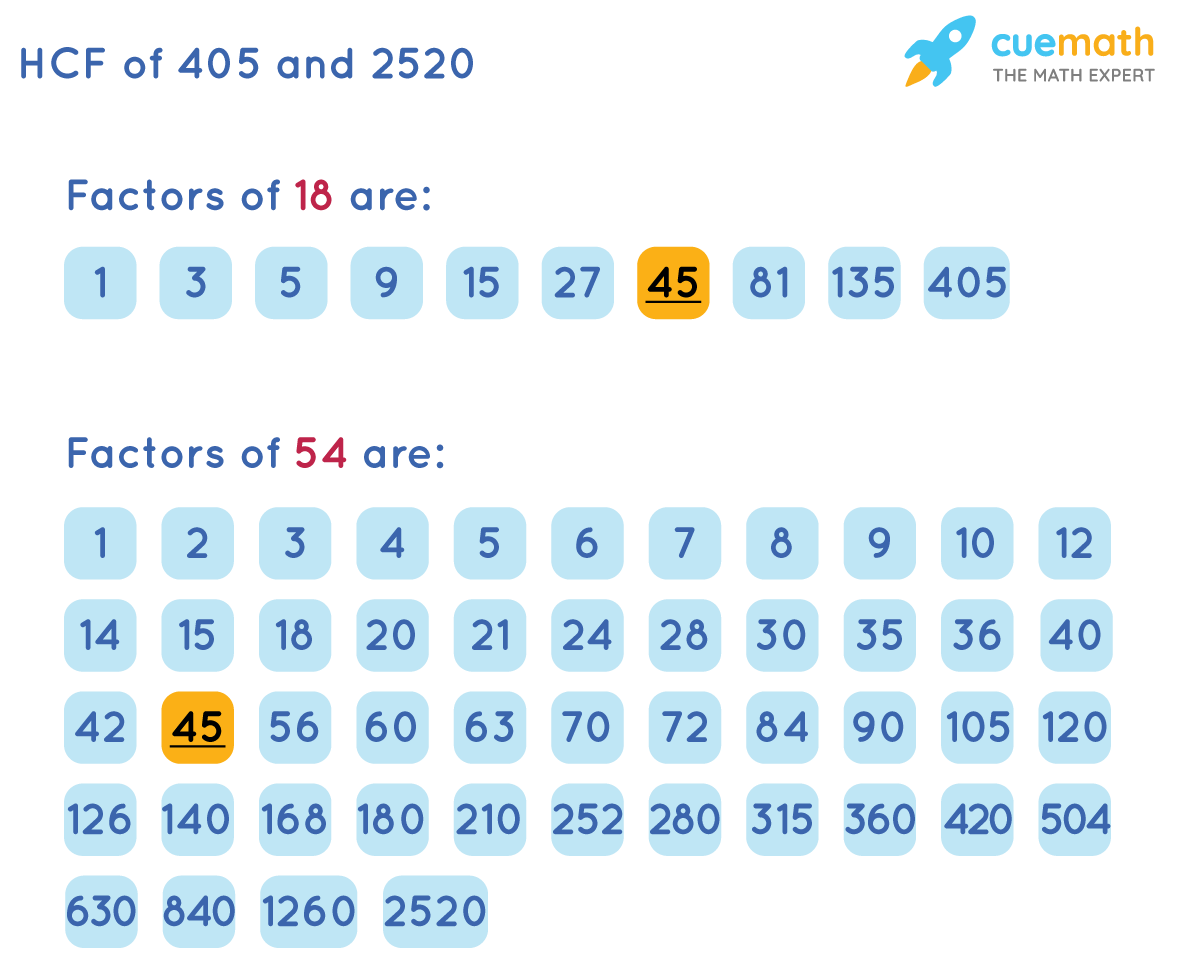# Find the HCF of 405 and 2520

## Question: Find the HCF of 405 and 2520?

The largest possible number which divides the given numbers exactly without any remainder is called the HCF (Highest Common Factor).

## Answer: HCF of 405 and 2520 is 45

We will explain two methods to find the HCF of 405 and 2520

## Explanation:

The two methods that we are using to find HCF of 405 and 2520 are shown below.

• HCF of 405 and 2520 by Prime Factorization
• HCF of 405 and 2520 by Listing the Common Factors

### Method 1: Find HCF of 405 and 2520 by Prime Factorization

Represent 405 and 2520 as a product of its prime factors.

Prime factorization of 405 is 3 × 3 × 3 × 3 × 5

Prime factorization of 2520 is 2 × 2 × 2 × 3 × 3 × 5 × 7

The common factor in the prime factorization of 405 and 2520 is  3 × 3 × 5

HCF is the product of the factors that are common to each of the given numbers.

So, HCF of 405 and 2520 is 45

### Method 2: Find HCF of 405 and 2520 by Listing the Common Factors

The factors of 405 and 2520 are shown below.The common factors of 405 and 2520, are, 1, 3, 5, 9, 15, and 45

The highest common factor of 405 and 2520 is 45

You can find the HCF in any of the above methods but the solution will be the same.